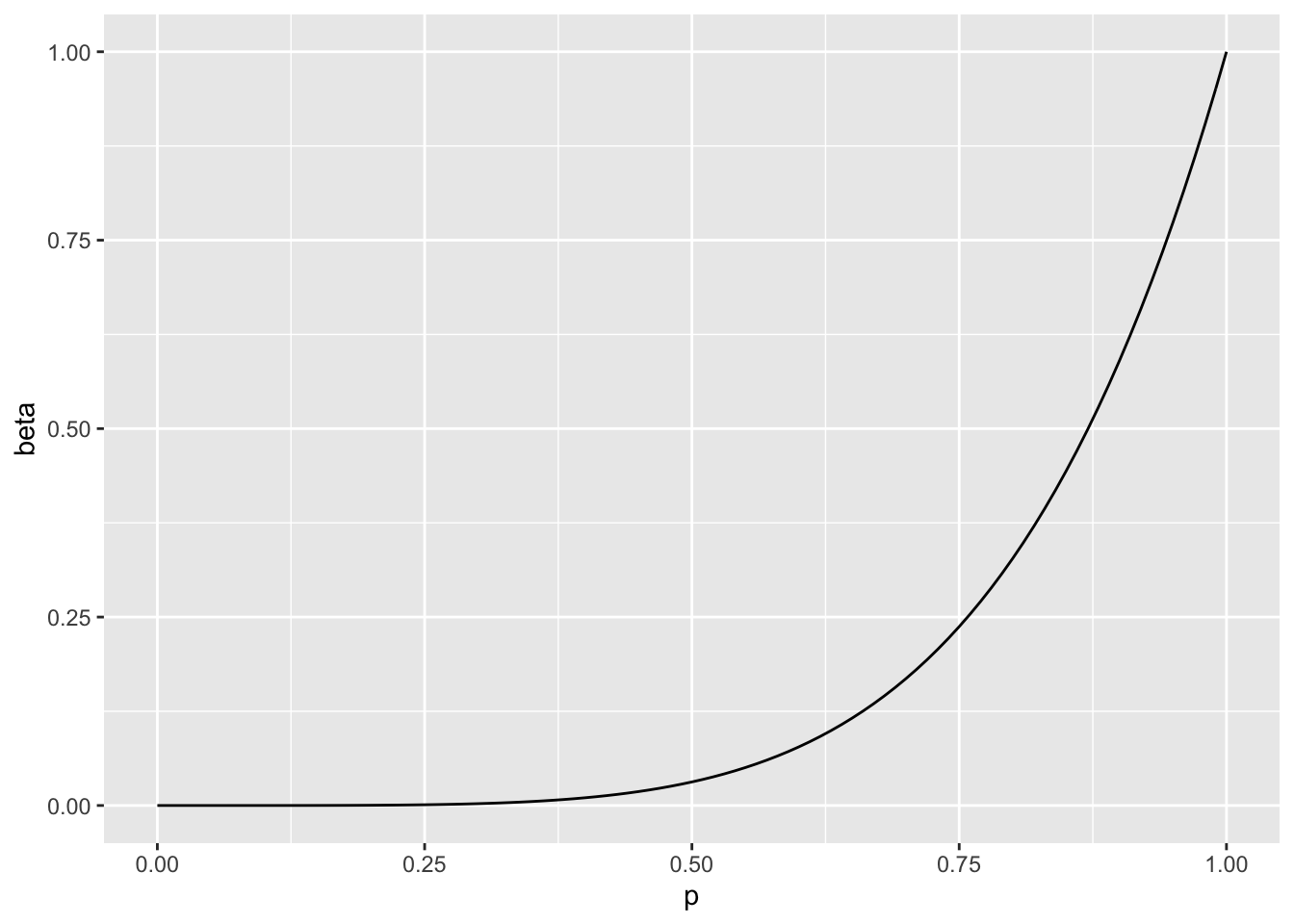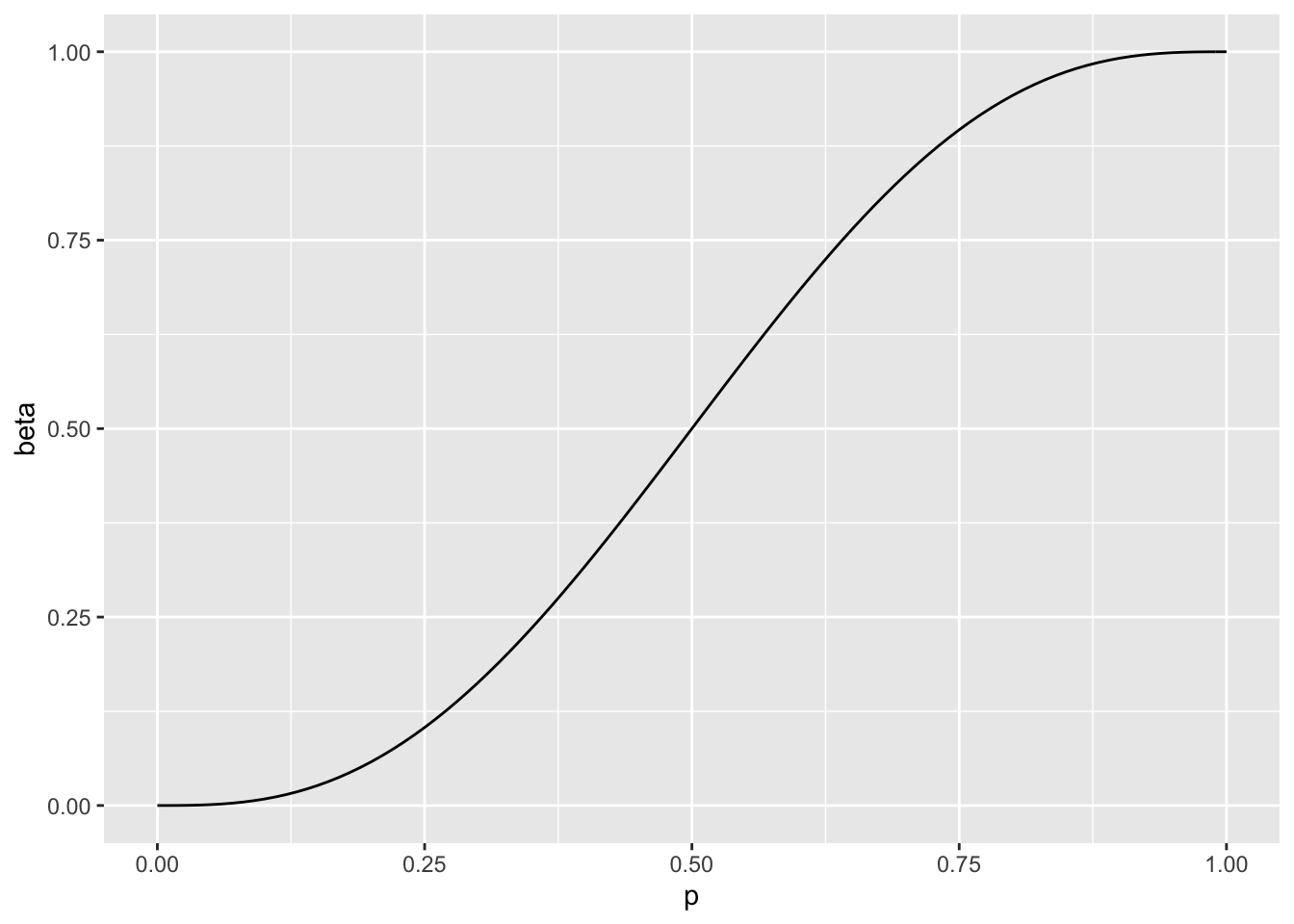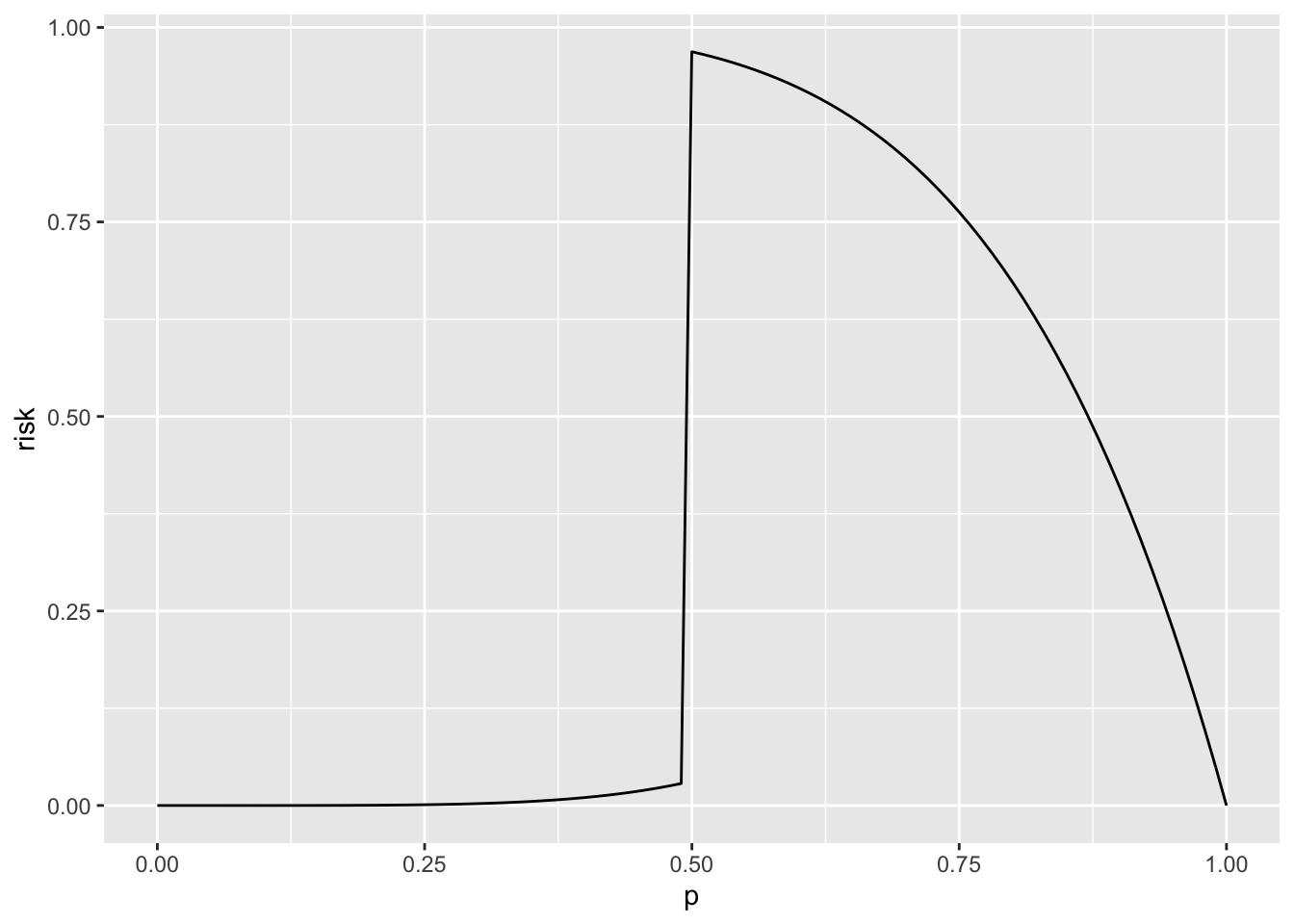## Definition of hypotheses

A hypothesis is a statement about a population parameter.

$H_0: \theta \in \Theta_0 \text{ and } H_1: \theta \in \Theta_1$

Often $$\Theta_1 = \Theta_0^C$$.

### Types of hypotheses

#### Simple hypothesis

$H_0: \theta = \theta_0$

#### Composite hypothesis

When the hypothesis is not simple.

### Types of hypothesis test

#### Simple

$H_0: \theta = \theta_0 \text{ and } H_1: \theta = \theta_1$

#### Composite

##### One-side

$H_0: \theta \leq \theta_0 \text{ and } H_1: \theta > \theta_0$

or

$H_0: \theta \geq \theta_0 \text{ and } H_1: \theta > \theta_0$

##### Two-sides

$H_0: \theta = \theta_0 \text{ and } H_1: \theta \neq \theta_0$

That is, under $$H_1$$, $$\theta = (\theta_1,\dotsc,\theta_m,\theta_{m+1},\dotsc,\theta_{m+p})$$ and under $$H_0$$, $$\theta = (\theta_1,\dotsc,\theta_m,\theta_{m+1}=\theta_{0,1},\dotsc,\theta_{m+p}=\theta_{0,p})$$.

## Action space

$$\mathcal{A} = \{ a_0, a_1\}$$ where $$a_0$$ represents choosing $$H_0$$ and $$a_1$$ represents choosing $$H_1$$

## Decision rule

In the context of hypothesis testing, a decision rule is called hypothesis test and it is defined by the sample values $$x$$ for which we accept or reject $$H_0$$. The sample values for which we reject $$H_0$$ is called the rejection region $$R$$.

$$d(x) = \left\{ \begin{array}{ll} a_0 \ if \ x \not\in R \\ a_1 \ if \ x \in R \\ \end{array} \right.$$

The rejection region is often specified by establishing a criterium on a test statistic $$W(x)$$ (function of the sample):

$R=\{x: W(x)<c\}$

A common method to find a test statistic is the likelihood ratio. To completely specify $$R$$, we need $$c$$ which can be obtained by setting the probabilities of making mistakes.

### Example

$$d(x) = \left\{ \begin{array}{ll} a_0 \ if \ \overline{x} \leq 4.3 \\ a_1 \ if \ \overline{x} > 4.3 \\ \end{array} \right.$$

## Evaluating tests (probabilities of making mistakes)

Type I error: $$H_0$$ is true $$(\theta \in \Theta_0)$$ and $$a=a_1$$.

Type II error: $$H_1$$ is true $$(\theta \in \Theta_1)$$ and $$a=a_0$$.

There are 4 interesting probabilities:

$$P(a_1 | H_0) = P (Type \,I\, error)$$

$$P(a_1 | H_1)$$

$$P(a_0 | H_0)$$

$$P(a_0 | H_1) = P (Type \, II \, error)$$

### Power function of a test (power function of a rejection region)

$\beta(\theta) = P_\theta (X \in R)$

A good test should have $$\beta(\theta)$$ near 0 when $$\theta \in \Theta_0$$ and near 1 when $$\theta \in \Theta_1$$.

#### Example 1

$$X \sim B(5,p)$$

$$H_0: p \leq 1/2$$

$$H_1: p > 1/2$$

$$R=\{k = 5 \}$$

$$\beta(p) = P_p(k \in R) = P_p(k = 5) = \binom{5}{5} p^5 (1-p)^0 = p^5$$

library(ggplot2)
## Warning in file(con, "r"): cannot open file '/var/db/timezone/zoneinfo/
## +VERSION': No such file or directory
p <- seq(0,1,.01)
beta <- p^5
dat <- data.frame(p,beta)
ggplot(dat,aes(x=p,y=beta))+geom_line()The probability of Type II error is quite large.

$$R=\{k \geq 3 \}$$

$$\beta(p) = P_p(k \in R) = P_p(k = 3 \ or \ k = 4 \ or \ k =5 ) = \binom{5}{3} p^3 (1-p)^2 + \binom{5}{4} p^4 (1-p)^1 + \binom{5}{5} p^5(1-p)^0$$

p <- seq(0,1,.01)
beta <- 10*p^3*(1-p)^2 + 5*p^4*(1-p)+p^5
dat <- data.frame(p,beta)
ggplot(dat,aes(x=p,y=beta))+geom_line()The Type II error decreases, but Type I increases.

### Size $$\alpha$$ of a test

$sup_{\theta \in \Theta_0} \beta(\theta) = \alpha$

In example 1, $$\alpha$$s are respectively

p <- .5
p^5
##  0.03125
10*p^3*(1-p)^2 + 5*p^4*(1-p)+p^5
##  0.5

### Level $$\alpha$$ of a test

$sup_{\theta \in \Theta_0} \beta(\theta) \leq \alpha$

### Null hypothesis testing

$$H_0$$ and $$H_1$$ are treated asymmetrically as $$\alpha$$ is fixed (often to .01 or .05) so that the Type I error is less than $$\alpha$$.

### UMP

A test in class $$\mathcal{C}$$ with a power function $$\beta(\theta)$$ is the uniformly most powerful (UMP) test of class $$\mathcal{C}$$ if $$\beta(\theta) \geq \beta'(\theta)$$ for all $$\theta \in \Theta_1$$.

#### UMP level $$\alpha$$

$$\mathcal{C} = \text{ all level } \alpha \text{ tests}$$.

### p-value

A p-value $$p(X)$$ is a test statistics that satisfies $0 \leq p(X) \leq 1$

It is valid if $P_{\theta} \left( p \left( X \right) \leq \alpha\right) \leq \alpha$

### Loss and risk functions

#### 0-1

$$L(\theta,a) = \left\{ \begin{array}{ll} 1 & \mbox{if } \theta \in \Theta_0 \text{ and } a=a_1\\ 1 & \mbox{if } \theta \in \Theta_1 \text{ and } a=a_0 \\ 0 & \text{otherwise} \\ \end{array} \right.$$

$$R(\theta,d) = \left\{ \begin{array}{ll} P_{\theta\ \in \Theta_0}\left(d(X)=a_1\right) =\beta(\theta) & \mbox{if } \theta \in \Theta_0 \\ P_{\theta\ \in \Theta_1}\left(d(X)=a_0\right) =1 -\beta(\theta) & \mbox{if } \theta \in \Theta_1 \\ \end{array} \right.$$

Demonstration

If $$\theta \in \Theta_0$$

$$R(\theta,d(X)) = \int_R L(\theta,d(X)) f(x; \theta) \, dx + \int_{R^C} L(\theta,d(X)) f(x; \theta) \, dx = \int_R L(\theta,a_1) f(x; \theta) \, dx + \int_{R^C} L(\theta,a_0) f(x; \theta) \, dx= \\ = \int_R 1 \, f(x; \theta) \, dx + \int_{R^C} 0 \, f(x; \theta) \, dx = \int_R f(x; \theta) \, dx = P_{\theta\ \in \Theta_0}(d(X)=a_1)$$

If $$\theta \in \Theta_1$$

$$R(\theta,d(X)) = \int_R L(\theta,d(X)) f(x; \theta) \, dx + \int_{R^C} L(\theta,d(X)) f(x; \theta) \, dx = \int_R L(\theta,a_1) f(x; \theta) \, dx + \int_{R^C} L(\theta,a_0) f(x; \theta) \, dx= \\ = \int_R 0 \, f(x; \theta) \, dx + \int_{R^C} 1 \, f(x; \theta) \, dx = \int_{R^C} f(x; \theta) \, dx = P_{\theta\ \in \Theta_1}(d(X)=a_0)$$

##### Example
p <- seq(0,1,.01)
risk <- ifelse(p<.5, p^5, 1 - p^5)
dat <- data.frame(p,risk)
ggplot(dat,aes(x=p,y=risk))+geom_line()#### Generalised 0-1

$$L(\theta,a) = \left\{ \begin{array}{ll} c_I & \mbox{if } \theta \in \Theta_0 \text{ and } a=a_1\\ c_{II} & \mbox{if } \theta \in \Theta_1 \text{ and } a=a_0 \\ 0 & \text{otherwise} \\ \end{array} \right.$$

$$R(\theta,d) = \left\{ \begin{array}{ll} P_{\theta\ \in \Theta_0}\left(d(X)=a_1\right) =c_I \beta(\theta) & \mbox{if } \theta \in \Theta_0 \\ P_{\theta\ \in \Theta_1}\left(d(X)=a_0\right) =c_{II} \left( 1 -\beta(\theta) \right) & \mbox{if } \theta \in \Theta_1 \\ \end{array} \right.$$

#### More general

$$L(\theta,a) = \left\{ \begin{array}{ll} -V_{00} & \mbox{if } \theta \in \Theta_0 \text{ and } a=a_0\\ V_{01} & \mbox{if } \theta \in \Theta_0 \text{ and } a=a_1\\ -V_{11} & \mbox{if } \theta \in \Theta_1 \text{ and } a=a_1\\ V_{10} & \mbox{if } \theta \in \Theta_1 \text{ and } a=a_0\\ \end{array} \right.$$

$$R(\theta,a) = \left\{ \begin{array}{ll} V_{10} P_{\theta\ \in \Theta_0}\left(d(X)=a_1\right) - V_{00} P_{\theta\ \in \Theta_0}\left(d(X)=a_0\right)& \mbox{if } \theta \in \Theta_0 \\ V_{01} P_{\theta\ \in \Theta_1}\left(d(X)=a_0\right) - V_{11} P_{\theta\ \in \Theta_1}\left(d(X)=a_1\right) & \mbox{if } \theta \in \Theta_1 \\ \end{array} \right.$$